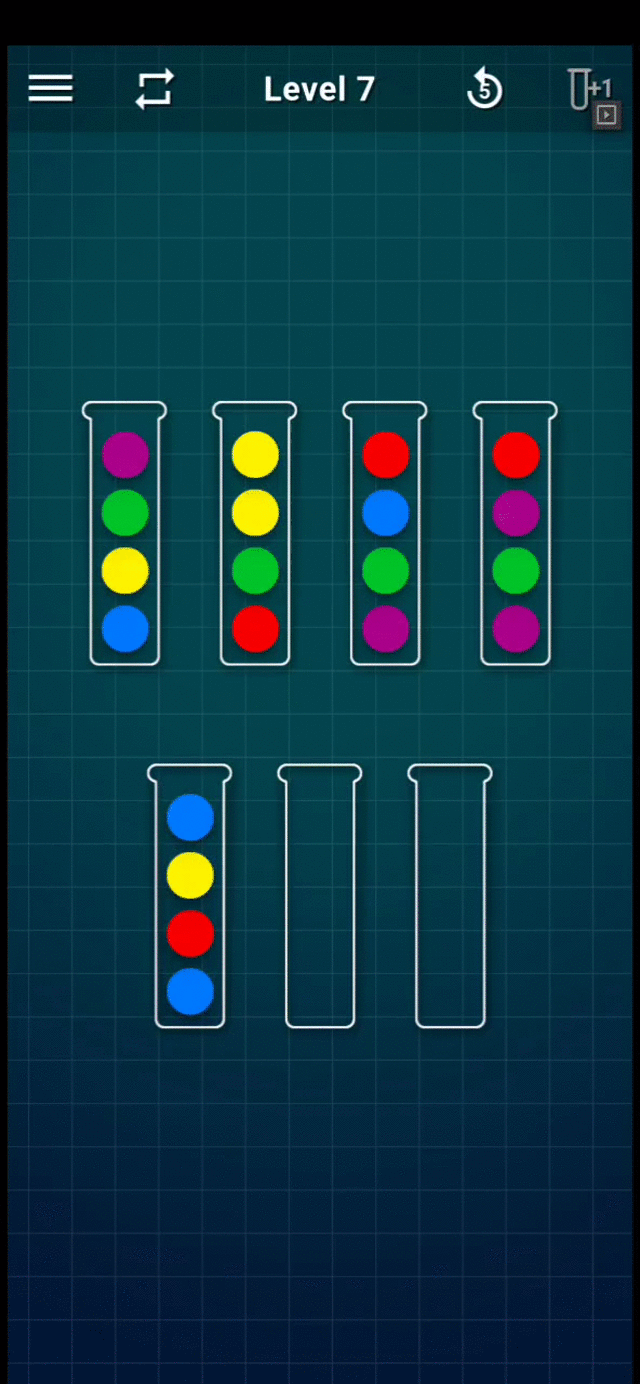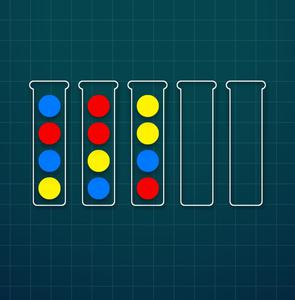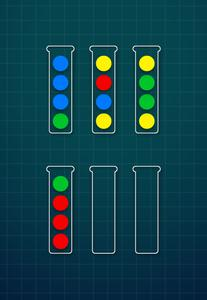Open in App
Not now

# Designing algorithm to solve Ball Sort Puzzle

• Difficulty Level : Hard
• Last Updated : 24 Mar, 2023

In Ball Sort Puzzle game, we have p balls of each colour and n different colours, for a total of p×n balls, arranged in n stacks. In addition, we have 2 empty stacks. A maximum of p balls can be in any stack at a given time. The goal of the game is to sort the balls by colour in each of the n stacks.

Rules:

• Only the top ball of each stack can be moved.
• A ball can be moved on top of another ball of the same colour
• A ball can be moved in an empty stack.

Refer to the following GIF for an example game play (Level-7):Level 7 Gameplay

Approach I [Recursion and BackTrack]:

• From the given rules, a simple recursive algorithm could be generated as below:
• Create an initial empty Queue.
• loop:
• If the current position is sorted:
• return
• else
• Enqueue all possible moves in a Queue.
• Dequeue the next move from the Queue.
• Go to loop.

However, the approach looks simple and correct, it has few caveats:

• Incorrect:
• We might end up in an infinite loop if there are >1 moves in the Queue which lead to the same position of balls.
• Inefficient:
• We might end up visiting the same position multiple times.

Thus, eliminating the above-mentioned bottlenecks would solve the issue.

Approach II [Memoization using HashMap]:

• Assumptions:
• We’ll represent ball positions as a vector of strings: {“gbbb”, “ybry”, “yggy”, “rrrg”}
• Create a set called Visited of <String> which will contain the visited positions as one long string.
• Create an empty vector for Answer which will store positions<a, b> of the tubes to move the top ball from tube a to and put it in tube b.
• Initialise grid with the initial settings of the balls.
• func solver(grid):
• loop over all the stacks (i):
• loop over all the stacks (j):
• If move i->j is valid, create newGrid with that move.
• if the balls are sorted in newGrid,
• return;
• if newGrid is NOT in Visited
• solver(newGrid)
• if solved:

Sample Game Input I:Level 3

Sample Input I:

```5
ybrb
byrr
rbyy```

Sample Output I:

```Move 1 to 4 1 times
Move 1 to 5 1 times
Move 1 to 4 1 times
Move 2 to 5 2 times
Move 1 to 2 1 times
Move 3 to 1 1 times
Move 1 to 2 1 times
Move 3 to 1 1 times
Move 2 to 1 3 times
Move 2 to 3 1 times
Move 3 to 4 1 times
Move 3 to 2 1 times
Move 2 to 4 1 times
Move 3 to 5 1 times```

Sample Game Input II:Level 5

Sample Input II:

```6
gbbb
ybry
yggy
rrrg```

Sample Output II:

```Move 1 to 5 3 times
Move 2 to 6 1 times
Move 3 to 6 1 times
Move 1 to 3 1 times
Move 2 to 1 1 times
Move 2 to 5 1 times
Move 2 to 6 1 times
Move 3 to 2 3 times
Move 3 to 6 1 times
Move 4 to 2 1 times
Move 1 to 4 1 times```

Refer to the below C++ implementation with the comments for the reference:

## C++

 `// C++ program for the above approach` `#include ` `using` `namespace` `std;` `using` `Grid = vector;`   `Grid configureGrid(string stacks[], ``int` `numberOfStacks)` `{`   `    ``Grid grid;` `    ``for` `(``int` `i = 0; i < numberOfStacks; i++)` `        ``grid.push_back(stacks[i]);`   `    ``return` `grid;` `}`   `// Function to find the max` `int` `getStackHeight(Grid grid)` `{` `    ``int` `max = 0;` `    ``for` `(``auto` `stack : grid)` `        ``if` `(max < stack.size())` `            ``max = stack.size();` `    ``return` `max;` `}`   `// Convert vector of strings to` `// canonicalRepresentation of strings` `string canonicalStringConversion(Grid grid)` `{` `    ``string finalString;` `    ``sort(grid.begin(), grid.end());` `    ``for` `(``auto` `stack : grid) {` `        ``finalString += (stack + ``";"``);` `    ``}` `    ``return` `finalString;` `}`   `// Function to check if it is solved` `// or not` `bool` `isSolved(Grid grid, ``int` `stackHeight)` `{`   `    ``for` `(``auto` `stack : grid) {` `        ``if` `(!stack.size())` `            ``continue``;` `        ``else` `if` `(stack.size() < stackHeight)` `            ``return` `false``;` `        ``else` `if` `(std::count(stack.begin(),` `                            ``stack.end(),` `                            ``stack)` `                 ``!= stackHeight)` `            ``return` `false``;` `    ``}` `    ``return` `true``;` `}`   `// Check if the move is valid` `bool` `isValidMove(string sourceStack,` `                 ``string destinationStack,` `                 ``int` `height)` `{`   `    ``// Can't move from an empty stack` `    ``// or to a FULL STACK` `    ``if` `(sourceStack.size() == 0` `        ``|| destinationStack.size() == height)` `        ``return` `false``;`   `    ``int` `colorFreqs` `        ``= std::count(sourceStack.begin(),` `                     ``sourceStack.end(),` `                     ``sourceStack);`   `    ``// If the source stack is same colored,` `    ``// don't touch it` `    ``if` `(colorFreqs == height)` `        ``return` `false``;`   `    ``if` `(destinationStack.size() == 0) {`   `        ``// If source stack has only` `        ``// same colored balls,` `        ``// don't touch it` `        ``if` `(colorFreqs == sourceStack.size())` `            ``return` `false``;` `        ``return` `true``;` `    ``}` `    ``return` `(` `        ``sourceStack[sourceStack.size() - 1]` `        ``== destinationStack[destinationStack.size() - 1]);` `}`   `// Function to solve the puzzle` `bool` `solvePuzzle(Grid grid, ``int` `stackHeight,` `                 ``unordered_set& visited,` `                 ``vector >& answerMod)` `{` `    ``if` `(stackHeight == -1) {` `        ``stackHeight = getStackHeight(grid);` `    ``}` `    ``visited.insert(` `        ``canonicalStringConversion(grid));`   `    ``for` `(``int` `i = 0; i < grid.size(); i++) {`   `        ``// Iterate over all the stacks` `        ``string sourceStack = grid[i];` `        ``for` `(``int` `j = 0; j < grid.size(); j++) {` `            ``if` `(i == j)` `                ``continue``;` `            ``string destinationStack = grid[j];` `            ``if` `(isValidMove(sourceStack,` `                            ``destinationStack,` `                            ``stackHeight)) {`   `                ``// Creating a new Grid` `                ``// with the valid move` `                ``Grid newGrid(grid);`   `                ``// Adding the ball` `                ``newGrid[j].push_back(newGrid[i].back());`   `                ``// Adding the ball` `                ``newGrid[i].pop_back();` `                ``if` `(isSolved(newGrid, stackHeight)) {` `                    ``answerMod.push_back(` `                        ``vector<``int``>{ i, j, 1 });` `                    ``return` `true``;` `                ``}` `                ``if` `(visited.find(` `                        ``canonicalStringConversion(newGrid))` `                    ``== visited.end()) {` `                    ``bool` `solveForTheRest` `                        ``= solvePuzzle(newGrid, stackHeight,` `                                      ``visited, answerMod);` `                    ``if` `(solveForTheRest) {` `                        ``vector<``int``> lastMove` `                            ``= answerMod[answerMod.size()` `                                        ``- 1];`   `                        ``// Optimisation - Concatenating` `                        ``// consecutive moves of the same` `                        ``// ball` `                        ``if` `(lastMove == i` `                            ``&& lastMove == j)` `                            ``answerMod[answerMod.size() - 1]` `                                     ``++;` `                        ``else` `                            ``answerMod.push_back(` `                                ``vector<``int``>{ i, j, 1 });` `                        ``return` `true``;` `                    ``}` `                ``}` `            ``}` `        ``}` `    ``}` `    ``return` `false``;` `}`   `// Checks whether the grid is valid or not` `bool` `checkGrid(Grid grid)` `{`   `    ``int` `numberOfStacks = grid.size();` `    ``int` `stackHeight = getStackHeight(grid);` `    ``int` `numBallsExpected` `        ``= ((numberOfStacks - 2) * stackHeight);` `    ``// Cause 2 empty stacks` `    ``int` `numBalls = 0;`   `    ``for` `(``auto` `i : grid)` `        ``numBalls += i.size();` `    ``if` `(numBalls != numBallsExpected) {` `        ``cout << ``"Grid has incorrect # of balls"` `             ``<< endl;` `        ``return` `false``;` `    ``}` `    ``map<``char``, ``int``> ballColorFrequency;` `    ``for` `(``auto` `stack : grid)` `        ``for` `(``auto` `ball : stack)` `            ``if` `(ballColorFrequency.find(ball)` `                ``!= ballColorFrequency.end())` `                ``ballColorFrequency[ball] += 1;` `            ``else` `                ``ballColorFrequency[ball] = 1;` `    ``for` `(``auto` `ballColor : ballColorFrequency) {` `        ``if` `(ballColor.second != getStackHeight(grid)) {` `            ``cout << ``"Color "` `<< ballColor.first` `                 ``<< ``" is not "` `<< getStackHeight(grid)` `                 ``<< endl;` `            ``return` `false``;` `        ``}` `    ``}` `    ``return` `true``;` `}`   `// Driver Code` `int` `main(``void``)` `{`   `    ``// Including 2 empty stacks` `    ``int` `numberOfStacks = 6;` `    ``std::string stacks[]` `        ``= { ``"gbbb"``, ``"ybry"``, ``"yggy"``, ``"rrrg"``, ``""``, ``""` `};`   `    ``Grid grid = configureGrid(` `        ``stacks, numberOfStacks);` `    ``if` `(!checkGrid(grid)) {` `        ``cout << ``"Invalid Grid"` `<< endl;` `        ``return` `1;` `    ``}` `    ``if` `(isSolved(grid, getStackHeight(grid))) {` `        ``cout << ``"Problem is already solved"` `             ``<< endl;` `        ``return` `0;` `    ``}` `    ``unordered_set visited;` `    ``vector > answerMod;`   `    ``// Solve the puzzle instance` `    ``solvePuzzle(grid, getStackHeight(grid),` `                ``visited,` `                ``answerMod);`   `    ``// Since the values of Answers are appended` `    ``// When the problem was completely` `    ``// solved and backwards from there` `    ``reverse(answerMod.begin(), answerMod.end());`   `    ``for` `(``auto` `v : answerMod) {` `        ``cout << ``"Move "` `<< v + 1` `             ``<< ``" to "` `<< v + 1` `             ``<< ``" "` `<< v << ``" times"` `             ``<< endl;` `    ``}` `    ``return` `0;` `}`

## Python3

 `def` `configureGrid(stacks, numberOfStacks):` `    `  `    ``grid ``=` `[]` `    ``for` `i ``in` `range``(numberOfStacks):` `        ``grid.append(stacks[i])` `    ``return` `grid`   `# Function to find the max` `def` `getStackHeight(grid):` `    ``max` `=` `0` `    ``for` `stack ``in` `grid:` `        ``if` `max` `< ``len``(stack):` `            ``max` `=` `len``(stack)` `    ``return` `max`   `# Convert vector of strings to` `# canonicalRepresentation of strings` `def` `canonicalStringConversion(grid):` `    ``finalString ``=` `""` `    ``grid.sort()` `    ``for` `stack ``in` `grid:` `        ``finalString ``+``=` `(stack ``+` `";"``)` `    ``return` `finalString`   `# Function to check if it is solved` `# or not` `def` `isSolved(grid, stackHeight):` `    ``for` `stack ``in` `grid:` `        ``if` `len``(stack) ``=``=` `0``:` `            ``continue` `        ``elif` `len``(stack) < stackHeight:` `            ``return` `False` `        ``elif` `stack.count(stack[``0``]) !``=` `stackHeight:` `            ``return` `False` `    ``return` `True`   `# Check if the move is valid` `def` `isValidMove(sourceStack, destinationStack, height):` `  `  `    ``# Can't move from an empty stack` `    ``# or to a FULL STACK` `    ``if` `len``(sourceStack) ``=``=` `0` `or` `len``(destinationStack) ``=``=` `height:` `        ``return` `False` `    `  `    ``colorFreqs ``=` `sourceStack.count(sourceStack[``0``])` `    `  `    ``# If the source stack is same colored,` `    ``# don't touch it` `    ``if` `colorFreqs ``=``=` `height:` `        ``return` `False` `    ``if` `len``(destinationStack) ``=``=` `0``:` `      `  `        ``# If source stack has only` `        ``# same colored balls,` `        ``# don't touch it` `        ``if` `colorFreqs ``=``=` `len``(sourceStack):` `            ``return` `False` `        ``return` `True` `    ``return` `sourceStack[``len``(sourceStack) ``-` `1``] ``=``=` `destinationStack[``len``(destinationStack) ``-` `1``]`   `# Function to solve the puzzle` `def` `solvePuzzle(grid, stackHeight, visited, answerMod):` `    ``if` `stackHeight ``=``=` `-``1``:` `        ``stackHeight ``=` `getStackHeight(grid)` `    ``visited.add(canonicalStringConversion(grid))` `    ``for` `i ``in` `range``(``len``(grid)):` `        ``# Iterate over all the stacks` `        ``sourceStack ``=` `grid[i]` `        ``for` `j ``in` `range``(``len``(grid)):` `            ``if` `i ``=``=` `j:` `                ``continue` `            ``destinationStack ``=` `grid[j]` `            ``if` `isValidMove(sourceStack, destinationStack, stackHeight):` `              `  `                ``# Creating a new Grid` `                ``# with the valid move` `                ``newGrid ``=` `list``(grid)` `                `  `                ``# Adding the ball` `                ``newGrid[j] ``+``=` `newGrid[i][``len``(newGrid[i]) ``-` `1``]` `                `  `                ``# Removing the ball` `                ``newGrid[i] ``=` `newGrid[i][:``-``1``]` `                ``if` `isSolved(newGrid, stackHeight):` `                    ``answerMod.append([i, j, ``1``])` `                    ``return` `True` `                ``if` `canonicalStringConversion(newGrid) ``not` `in` `visited:` `                    ``if` `solvePuzzle(newGrid, stackHeight, visited, answerMod):` `                        ``lastMove ``=` `answerMod[``len``(answerMod) ``-` `1``]` `                        `  `                        ``# Optimisation - Concatenating` `                        ``# consecutive moves of the same` `                        ``# ball` `                        ``if` `lastMove[``0``] ``=``=` `i ``and` `lastMove[``1``] ``=``=` `j:` `                            ``answerMod[``len``(answerMod) ``-` `1``][``2``] ``+``=` `1` `                        ``else``:` `                            ``answerMod.append([i, j, ``1``])` `                        ``return` `True` `    ``return` `False`   `# Checks whether the grid is valid or not` `def` `checkGrid(grid):` `    ``numberOfStacks ``=` `len``(grid)` `    ``stackHeight ``=` `getStackHeight(grid)` `    ``numBallsExpected ``=` `((numberOfStacks ``-` `2``) ``*` `stackHeight)` `    ``# Cause 2 empty stacks` `    ``numBalls ``=` `0` `    ``for` `i ``in` `grid:` `        ``numBalls ``+``=` `len``(i)` `    ``if` `numBalls !``=` `numBallsExpected:` `        ``print``(``"Grid has incorrect # of balls"``)` `        ``return` `False` `    ``ballColorFrequency ``=` `{}` `    ``for` `stack ``in` `grid:` `        ``for` `ball ``in` `stack:` `            ``if` `ball ``in` `ballColorFrequency:` `                ``ballColorFrequency[ball] ``+``=` `1` `            ``else``:` `                ``ballColorFrequency[ball] ``=` `1` `    ``for` `ballColor ``in` `ballColorFrequency:` `        ``if` `ballColorFrequency[ballColor] !``=` `getStackHeight(grid):` `            ``print``(``"Color"``, ballColor, ``"is not"``, getStackHeight(grid))` `            ``return` `False` `    ``return` `True`   `# Driver Code` `if` `__name__ ``=``=` `"__main__"``:` `    ``# Including 2 empty stacks` `    ``numberOfStacks ``=` `6` `    ``stacks ``=` `[``"gbbb"``, ``"ybry"``, ``"yggy"``, ``"rrrg"``, "``", "``"]` `    ``grid ``=` `configureGrid(stacks, numberOfStacks)` `    ``if` `not` `checkGrid(grid):` `        ``print``(``"Invalid Grid"``)` `        ``exit()` `    ``if` `isSolved(grid, getStackHeight(grid)):` `        ``print``(``"Problem is already solved"``)` `        ``exit()` `    ``visited ``=` `set``()` `    ``answerMod ``=` `[]` `    ``# Solve the puzzle instance` `    ``solvePuzzle(grid, getStackHeight(grid), visited, answerMod)` `    ``# Since the values of Answers are appended` `    ``# When the problem was completely` `    ``# solved and backwards from there` `    ``answerMod.reverse()` `    ``for` `v ``in` `answerMod:` `        ``print``(``"Move"``, v[``0``] ``+` `1``, ``"to"``, v[``1``] ``+` `1``, v[``2``], ``"times"``)`

Output

```Move 1 to 5 3 times
Move 2 to 6 1 times
Move 3 to 6 1 times
Move 1 to 3 1 times
Move 2 to 1 1 times
Move 2 to 5 1 times
Move 2 to 6 1 times
Move 3 to 2 3 times
Move 3 to 6 1 times
Move 4 to 2 1 times
Move 1 to 4 1 times```

Time Complexity: O(n!) where n is the number of stacks.

Auxiliary Space: O(n^2)

My Personal Notes arrow_drop_up
Related Articles